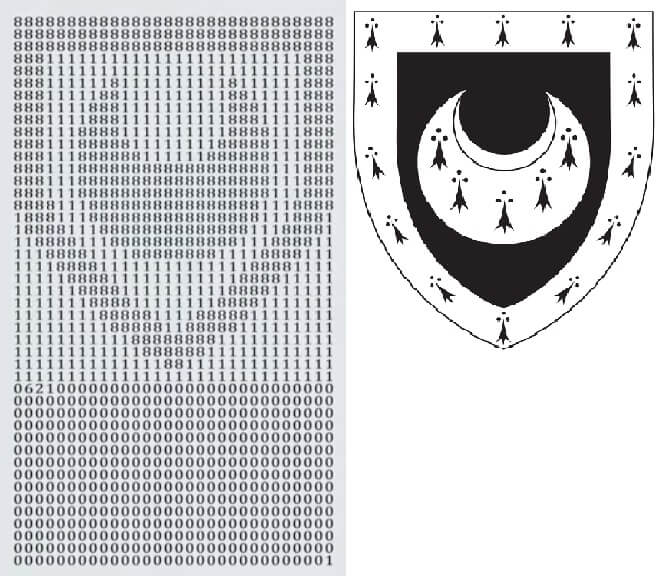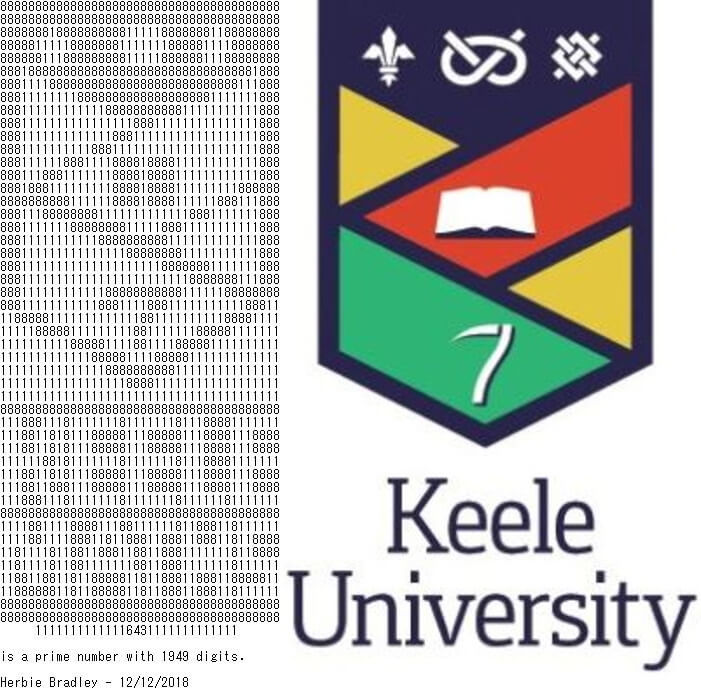# The Keele University Prime

December 18, 2018

Last year, the YouTube channel Numberphile published a video showing a prime number of 1350 digits that looks like the coat of arms of Trinity Hall College, Cambridge. The number 1350 is significant, since it is the year the college was founded.After coming across this video, I decided to try and make a similar number for Keele University (founded in 1949):## How did I make this?

I found several scripts around the internet to generate prime numbers from pictures, but none of them gave a good looking result so I decided to make the logo art manually. I estimated that the best size for the number was 40 columns x 48 rows, leaving a 49th row of 13 digits to make up the total of 1949 digits. Then I spent about four hours flipping the digits to get the best looking logo.

Next, I wrote a Python script to iterate over three digits in the middle of the bottom row and test each possibility with the Miller-Rabin probabilistic primality test. On my laptop, this computation took only a few minutes to check all 1000 combinations, and returned one possible number which was very likely to be prime. Finally, I used the tool on Dario Alpern’s website to make sure that the Miller-Rabin test was correct, and the number actually was prime.

I also tried iterating over a few other three digit sequences in the number and found several primes there too, but I decided the prime shown above looked the best. The script I used is here.

## How does this work?

By the prime number theorem, there are approximately $$\frac{n}{\log n}$$ primes less than $$n$$. Hence there are approximately

$\frac{10^{1949}}{\log 10^{1949}} - \frac{10^{1948}}{\log 10^{1948}} \approx 2.005 \times 10^{1945}$

primes of 1949 digits. There are $$4.5 \times 10^{1948}$$ odd numbers of 1949 digits, so approximately one in every 2244 numbers I check is prime. This is why iterating over a three digit sequence is a reasonable thing to do, since you are quite likely to hit a prime in the first or second three digit sequence you try. In the Trinity Hall prime you can see a place where the author also looped over a sequence of three digits.